# In-flight Correction of the Satellite Orientation Parameter during Target Mode

In-flight Correction of the Satellite Orientation Parameter during Target Mode

Amr A. AliMoutaz M. Hegaze Ahmed S. Elrodesly

Department of Engineering Mechanics, Military Technical College, Cairo 11838, Egypt

Faculty of Applied Science and Engineering Technology, North York M2J2X5, Canada

Corresponding Author Email:
amraali.eg@gmail.com
Page:
249-262
|
DOI:
https://doi.org/10.18280/mmep.060213
25 November 2018
|
Accepted:
19 March 2019
|
Published:
20 June 2019
| Citation

OPEN ACCESS

Abstract:

Spacecraft attitude and orbit control systems have different levels of modes; complex, function, and orientation modes. For earth remote sensing satellites, the orbital and target orientation modes are designed to create and maintain their attitude during execution of their main mission. They should guarantee image quality requirements. In this paper, a complete kinematics of fixed ground target tracking with preparation of the needed parameters for pointing correction has been presented. The desired orientation parameters related to such modes are calculated for the current time and forecasted for the instant of imaging start according to the flight task arrays. The relative velocity between the on-ground scene pattern and the satellite is calculated and used to determine the required correction of orientation parameters to enhance the image quality and pointing accuracy. The detailed steps of determination of such parameters are illustrated. A time-optimal control quaternion based algorism is used and modified to achieve the required agility performance using the maximum system capability through the maneuver direction. It is shown by in-flight case study that the proposed algorithm with the prepared correction actions achieve the required pointing accuracy and time efficiency, and maintain the system within the permissible limits.

Keywords:

orientation parameters, pointing accuracy, satellite attitude and orbit control, time-optimal nonlinear feedback control

1. Introduction

The attitude and orbit control (AOC) of any satellite, or, in general any spacecraft (SC), is performed in a number of modes which can be classified from a different points of views. From the SC state point of view, SC may be in transfer, initial, operating, or survival mode. In the transfer mode, SC angular rate is controlled and damped. For initial mode, SC initial attitude is determined and the desired initial orientation is settled. Survival mode, coarse control, is a safe mode implemented under safety aspect. Operating mode, fine control, is the normal working mode with the best performance and precise orientation. The operation of the AOC system may be organized to several levels of modes; complex, functional, service and orientation modes. The orientation mode may be; damping, inertial orientation, orbital orientation, 3-axis stabilized sun orientation, one-axis sun orientation, current inertial orientation, target orientation, spin by the velocity, instrumental bases spin, or turn mode. Almost, imaging and downloading as SC main mission for earth remote sensing satellites are executed based on orbital or target mode. Generally, SC attitude control is performed as closed loop control. The actual attitude is determined and compared with the desired to generate the control error. That is the input of the controller, while the control torque vector which desired to produce along the three SC-body-axes is the controller output.  The actuators should be able to produce the demanded torque in order to achieve the desired attitude. Attitude control aim is to achieve and keep the desired orientation parameters, attitude quaternion and angular rate, corresponding to the selected mode. The objective may be certain attitude and/or attitude rate.

In both cases, it is required to bring the attitude and angular rate errors to zero within certain tolerances. Correction of such orientation parameters during target mode is essential action to achieve the required pointing accuracy and imaging quality. The control law as a cascade saturation logic  has been widely used for large-angle fast acquisition and pointing maneuvers. For a given rotational maneuver with certain initial and final states of attitude and angular rate, the instantaneous variable limit of angular rate is determined based on the maneuver braking curve . This curve depends on the control capability of the system . Generally, it contains acceleration and deceleration phases. By the end of the deceleration phase, the desired SC attitude and attitude rate should be reached at a specified safe angular rate and angular deceleration achieving the required stabilization and pointing accuracy free of chattering problem .

The SC equations of motion are formulated as coupled SC orbit and attitude equations  in order to achieve the accepted accuracy which meets the requirements of the designed complex modes ; multi-targets tracking and orbit correction. This paper presents in detail the calculation of the quaternion based desired orientation parameters; attitude quaternion and angular rate, related to such modes. These parameters are calculated onboard instantaneously and forecasted for the instant of imaging start according to the preplanned flight task arrays. The desired orientation parameters are corrected based on the estimated state vector at imaging start instant. This correction enhances the pointing accuracy.

The following sections are organized as follows: Section II explores the basic kinematic and dynamic relations related to this topic. Section III shows the used modified quaternion based time-optimal control. Section IV introduces the orbital orientation mode and obtains the orbital quaternion and angular rate. In section V, the target orientation mode is introduced, geodetic coordinate system is utilized, and the desired quaternion and angular rate are calculated. Section VI illustrates the results of in-flight case study. Section VII illustrates the Euler angles representation for the desired and actual orientation parameters and the calculated attitude and attitude rate errors. Section VIII illustrates the controller limits comparison. Finally, section IX summarizes the results and conclusion.

2. SC Attitude Dynamics

2.1 Coordinate systems

The used right-handed coordinate systems are defined as :

1. Earth-Centered Inertial Coordinate System (ECI): It can be defined as Inertial Coordinate System (ICS), with standard epoch J2000, +X axis refers to the spring mean equilibrium point, and +Z axis directs to the north celestial pole.
2. Earth-Centered Earth-Fixed (ECEF) Coordinate System: It rotates with the Earth: with +X axis points in the direction of the Earth’s prime meridian, +Z axis refers to the Earth-pole, +Y axis completes right hand coordinate system and lies in plane of geographic equator, and the rotation angle is known as the Greenwich Mean Sidereal Time (GMST) angle.
3. Orbital Coordinate System (OCS): It is centered in the SC mass center: with +Y axis is directed along radius-vector to the center of the Earth, and +X coincides with vector of orbital angular rate.
4. Target (Object) Coordinate System (OCS-N): It is centered in the SC mass center: with +Y axis is directed toward the object of imaging, and the projection of axis -Z is perform acute angle with the direction of the vector of orbital angular rater (angle between -Z axis and orbit plane is equal to the required additional turn angle in yaw to prevent smeared picture).

2.2 Representation in different coordinate systems

The SC state vector in the ECI system  can be roughly converted to  in the ECEF system as follows 

$\left[\mathrm{r}_{\mathrm{ECEF}}\right]=\left[A_{G I}\right]\left[\mathrm{r}_{\mathrm{ECI}}\right]$ (1)

where $\left[A_{G I}\right]$ is the transformation matrix given by

$A_{G I}=\left[\begin{array}{ccc}{\cos \theta_{G}} & {\sin \theta_{G}} & {0} \\ {-\sin \theta_{G}} & {\cos \theta_{G}} & {0} \\ {0} & {0} & {1}\end{array}\right]$ (2)

$\theta_{G}$, as illustrated in Figure 1, is the Greenwich sidereal time at a specific epoch

$\theta_{G}=\theta_{G 0}+\omega_{e} t$(3)

$\theta_{G O}$ indicates the Greenwich sidereal time at 00:00:00 UT, $\omega_{e}=7.2921151467 e^{-5}[r a d / s]$ is the inertial rotation rate of the Earth, and is the time duration since 0 hours UT.

## figure_1.jpg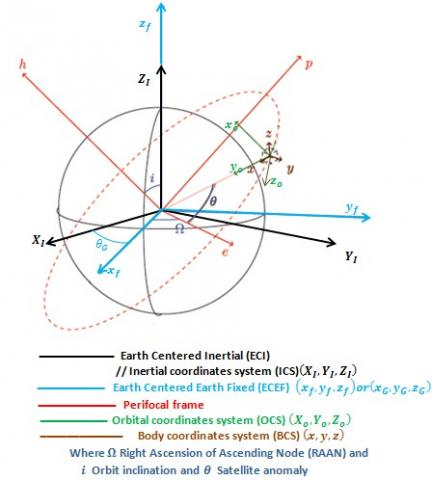Figure 1. Reference coordinate systems

## figure_2.jpg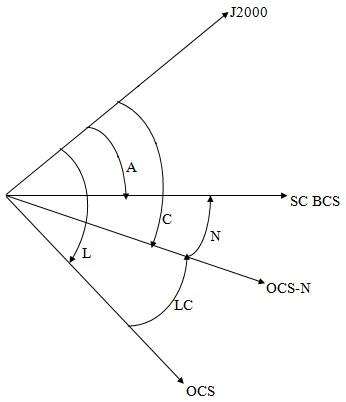Figure 2. Quaternion representation

Table 1. Quaternion description

 Abbreviation Quaternion description A Quaternion of transition from ICS to BCS (orientation of BCS relative to ICS). C Target quaternion, Quaternion of transition from ICS to target, (orientation of target relative to ICS). L Calculated orbital quaternion of transition from ICS to OCS, using navigation state vector, (orientation of orbital coordinate system relative to ICS). LC Target quaternion, Quaternion of transition from OCS to target, (orientation of target relative to OCS). N Control quaternion, which represents mismatch quaternion between desired (calculated) orientation and current BCS orientation.

2.3 SC attitude dynamics

By observing the change of attitude matrix over time, the kinematic equation can be derived as a relation between the attitude and angular rates. Using the quaternion form the kinematics relations are summarized as follows

$\dot{q}_{v e c}=-(1 / 2) \Omega q_{v e c}+(1 / 2) q_{4} \omega$

$\dot{q}_{4}=-(1 / 2) \omega^{T} q_{v e c}$(4)

where $\omega=\omega_{b I}=\left[\omega_{1}, \omega_{2}, \omega_{3}\right]^{T}$ is the SC absolute angular velocity, relative to the inertial reference frame (I), projected into the SC body coordinate system (b) and measured by rate gyros. The components of the quaternions q are defined as

$q_{1}=e_{x} \sin (\emptyset / 2)$

$q_{2}=e_{y} \sin (\emptyset / 2)$ (5)

$q_{3}=e_{z} \sin (\emptyset / 2)$

$q_{4}=\cos (\emptyset / 2)$

where $e_{x}, e_{y}, e_{z}$ are the components of the Euler axis unit vector in the reference frame. $\varnothing$ is the rotation angle around the Euler axis. From equation (5), it is clear that the quaternion components satisfy the following constraint

$q_{1}^{2}+q_{2}^{2}+q_{3}^{2}+q_{4}^{2}=1$(6)

In general, the quaternion q can be defined as $q=q_{s c a l}+q_{v e c}$ where:

$q_{s c a l}=q_{4}$ is the scalar part of the quaternion q,

$q_{v e c}=\left[q_{1}, q_{2}, q_{3}\right]^{T}$ is the vector part of the quaternion q.

The transformation of any vector from the reference frame (I) to the body frame (b) can be performed using the transformation matrix $A_{b I}$, which can be written in terms of quaternion components as 

$A_{b l}=\left[\begin{array}{ccc}{q_{1}^{2}-q_{2}^{2}-q_{3}^{2}+q_{4}^{2}} & {2\left(q_{1} q_{2}+q_{3} q_{4}\right)} & {2\left(q_{1} q_{3}-q_{2} q_{4}\right)} \\ {2\left(q_{1} q_{2}-q_{3} q_{4}\right)} & {-q_{1}^{2}+q_{2}^{2}-q_{3}^{2}+q_{4}^{2}} & {2\left(q_{2} q_{3}+q_{1} q_{4}\right)} \\ {2\left(q_{1} q_{3}+q_{2} q_{4}\right)} & {2\left(q_{2} q_{3}-q_{1} q_{4}\right)} & {-q_{1}^{2}-q_{2}^{2}+q_{3}^{2}+q_{4}^{2}}\end{array}\right]$ (7)

Equation (7) can be represented using various attitude parameters. In general, the descriptions of SC attitude can be given via quaternion or Euler angles parameters. Given Euler angles, a unique orientation is defined. Given orientation, non-unique Euler angles can be determined. Although the Euler angles representation has a clear physical interpretation in the roll, pitch and yaw angles, it unfortunately suffers from singularities. The quaternion representation has no singularities and no trigonometric functions.

The dynamic equation of motion is introduced by Euler equation and can be summarized as follows

$J \dot{\omega}=-\omega \times(J \omega)+M$ (8)

$\dot{\omega}=J^{-1}(-\Omega(\omega) J \omega+M)$(9)

$M=u+T_{d}$(10)

where J is the SC inertia matrix, M is the projection of the total applied moments about the SC centroid principal axes, $u=\left[u_{1}, u_{2}, u_{3}\right]^{T}$ is the control torque vector, $T_{d}$ is the disturbance torques and $\Omega(\omega)$ is a skew-symmetric matrix defined by

$\Omega(\omega)=\left[\omega^{X}\right]=\left[\begin{array}{ccc}{0} & {-\omega_{3}} & {\omega_{2}} \\ {\omega_{3}} & {0} & {-\omega_{1}} \\ {-\omega_{2}} & {\omega_{1}} & {0}\end{array}\right]$(11)

3. Quaternion Based Time-Optimal Control

Practically, the direct implementation of ideal time-optimal switching control logic tends to chattering problem due to time delays in the control system and various uncertainties . The uncertainties can be in the moment of inertia J and/or actuators dynamics where the maximum actuator torque is not exactly known. To eliminate this effect, a conventional linear control solution will replace the bang-bang solution near the origin.

3.1 Linear quaternion feedback control

In order to achieve the Eigen-axis rotations during the linear range, at the end of maneuver, a linear state feedback controller is used as follows 

$u=-K e-D e_{\omega}-\mu \Omega J \omega$(12)

Where $\mathrm{e}=\left[q_{1 e}, q_{2 e}, q_{3 e}\right]^{T}$ is the vector part of attitude error quaternion $q_{e}$ between the current orientation $q$ and the desired orientation $\mathcal{q}_{c}$, and defined as follows

$\left[\begin{array}{c}{q_{1 e}} \\ {q_{2 e}} \\ {q_{3 e}} \\ {q_{4 e}}\end{array}\right]=\left[\begin{array}{cccc}{q_{4 c}} & {q_{3 c}} & {-q_{2 c}} & {-q_{1 c}} \\ {-q_{3 c}} & {q_{4 c}} & {q_{1 c}} & {-q_{2 c}} \\ {q_{2 c}} & {-q_{1 c}} & {q_{4 c}} & {-q_{3 c}} \\ {q_{1 c}} & {q_{2 c}} & {q_{3 c}} & {q_{4 c}}\end{array}\right]\left[\begin{array}{c}{q_{1}} \\ {q_{2}} \\ {q_{3}} \\ {q_{4}}\end{array}\right]$ (13)

lternatively, it can define as

$q_{e}=\tilde{q}_{c} \circ q$(14)

The shaped angular rate error vector $e_{\omega}$ is calculated as follows

$e_{\omega}=\omega^{B C S}-\omega_{d e s}^{B C S}$(15)

where $\omega^{B C S}$ is the measured SC absolute angular velocity vector projected into BCS and $\omega_{d e s}^{B C S}$ is the desired angular velocity vector calculated according to the orientation mode then projected into BCS.

The feedback controller for Eigen-axis rotations consists of linear attitude error feedback $-K e$ and linear body-rate error feedback $-D e_{\omega}$. In order to counteract the effect of the gyroscopic coupling torque a nonlinear body-rate feedback term $-\mu \Omega J \omega$ can be added. Where μ=1 means that the control torque exactly counteracts the gyroscopic coupling torque, while $\mu=0$ means that only quaternion feedback and linear rate feedback are used. This controller is a time invariant closed loop controller in which no reference trajectory is calculated in advance for the mid-time until reaching the required final attitude, and the control at any time is calculated as a function of the current measured attitude and angular rate without any use of time dependent gains or functions. The gains K and D are 3X3 controller gain matrices should be properly determined or designed. These gains are selected according to Wie  as: $D=d J$ and $K=k J$, where d and k are scalars for the Eigen-axis rotation.

3.2 Time-optimal control

For fast orientation within specified maximum slew rate, the control torque input u can be calculated using the following saturation control logic 

$u=-J\left\{2 k_{L i}^{s a t}(e)+d e_{\omega}\right\}$(16)

For achieving rapid transient settlings for large attitude-error signals, the attitude error saturation limits $L_{i}$ are determined following  as

$L_{i}=(d / 2 k) \min \left\{\sqrt{4 a_{i}\left|e_{i}\right|},\left|\omega_{i}\right|_{\max }\right\}$(17)

where $\left|\omega_{i}\right|_{\max }$ is the maximum angular rate about each control axis, $a_{i}=U / J_{i i}$ is the maximum control acceleration components, and U is the same saturation limit for each control channel. The control torque is constrained symmetrically in 3 axes as follows

$-U \leq u_{i}(t) \leq+U \qquad i=1,2,3$(18)

3.3 Modified time-optimal control

In order to achieve the agility performance and guarantee the largest magnitudes of body rate and acceleration along the worst direction, different saturation limits $U=\left[U_{1}, U_{2}, U_{3}\right]^{T}$ are utilized [7-11]. The maximum control acceleration components in equation (17) are initially modified to $a_{i}=U_{i} / J_{i i}$ and an alternative definition of the normalized saturation function is used as follows

$\underset{\sigma}{a t}(u)=\left\{\begin{array}{ccc}{u} & {\text { if }} & {\sigma \leq 1} \\ {u / \sigma} & {\text { if }} & {\sigma>1}\end{array}\right\}$(19)

where

$\sigma=\|X u\|_{\infty}=\max \left\{|X u|_{i}\right\}$ (20)

$X=\operatorname{diag}\left(1 / U_{1}, 1 / U_{2}, 1 / U_{3}\right)$ (21)

Practically, the maximum torque which can be achieved in each control channel $U=\left[U_{1}, U_{2}, U_{3}\right]^{T}$ cannot be achieved at the same moment. So, the corresponding maximum control acceleration components $a_{e i}$ in each control axis are not freely independent and it can be calculated using the quaternion-error vector $e=\left(e_{1}, e_{2}, e_{3}\right)$ as follows

$a_{e i}=a_{\operatorname{maxp}} p_{i}$ (22)

$a_{\operatorname{maxp}}=\frac{1}{\sqrt{\frac{p_{1}^{2}}{a_{1}^{2}}+\frac{p_{2}^{2}}{a_{2}^{2}}+\frac{p_{3}^{2}}{a_{3}^{2}}}}$(23)

where $a_{m a x p}$ is the magnitude of the maximum control acceleration in the maneuver direction and $\widehat{P}=\left[p_{1}, p_{2}, p_{3}\right]^{T}$ is the Eigen-axis unit vector in the maneuver direction calculated by

$\hat{P}=\left\{\begin{array}{ccc}{-\frac{\operatorname{sgn}(e)}{\sqrt{3}}} & {\text { if }} & {\|e\| \leq \varepsilon} \\ {-\frac{e}{\|e\|}} & {\text { if }} & {\|e\|>\varepsilon}\end{array}\right\}$ (24)

where $\varepsilon$ is small positive number in order of $1^{-4}$ defining the final part of maneuver. Figure 3 illustrates the torque envelope of octahedron pyramid configuration reaction wheels cluster in comparison with the corresponding outer and inscribed ellipsoids. The maximum control acceleration $a_{e}$ is determined in the maneuver direction corresponding to the maximum (outer) torque ellipsoid defined by $U=\left[U_{1}, U_{2}, U_{3}\right]^{T}$. Due to the uncertainty in the torque generation, $a_{e}$ value should be reduced corresponding to the inscribed torque ellipsoid, while, the saturation limits stay corresponding to the outer torque ellipsoid.

Figure 3 illustrates that the maximum torque (outer) ellipsoid, contains unreachable torque values larger than the envelope capacity. Also it illustrates the unused portion from the torque envelope in case of inscribed ellipsoid. The attitude error saturation limits $L_{i}$ that are defined previously in equation (17) are redefined as

$L_{i}=(d / 2 k) \min \left\{\dot{e}_{e i},\left|\omega_{i}\right|_{\max }\right\}$(25)

where the constrained attitude rate $\dot{\Theta}_{\mathrm{ei}}$ is defined as

$\dot{e}_{e i}=\sqrt{\frac{4\left|e_{i}\right|\left|p_{i}\right|}{\sqrt{\frac{p_{1}^{2}}{a_{e 1}^{2}}+\frac{p_{2}^{2}}{a_{e 2}^{2}}+\frac{p_{3}^{2}}{a_{e 3}^{2}}}}}$ (26)

## figure_3a.png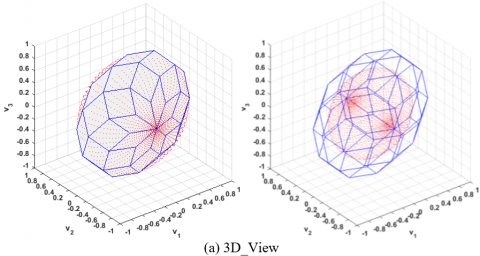## figure_3b.png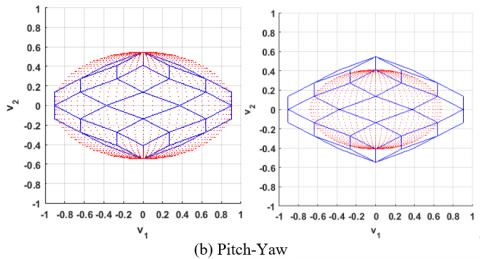## figure_3c.png## figure_3d.png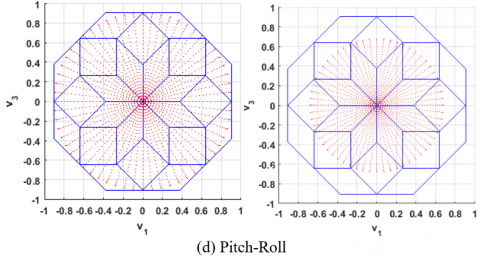Figure 3. 8-wheel outer & inscribed ellipsoids

Alternatively, it may be defined using Bailey patent  as

$\dot{e}_{e i}=\sqrt{\frac{4 e_{i}^{2}}{\sqrt{\frac{e_{1}^{2}}{a_{e 1}^{2}}+\frac{e_{2}^{2}}{a_{e 2}^{2}}+\frac{e_{3}^{2}}{a_{e 3}^{2}}}}}$ (27)

The control torque input u can be calculated using the saturation control logic shown in (16) and limited using the normalized saturation function shown in (20). However, equation (21) is modified as follows

$X=\operatorname{diag}\left(1 / U_{e 1}, 1 / U_{e 2}, 1 / U_{e 3}\right)$ (28)

where $U_{e}=\left[U_{e 1}, U_{e 2}, U_{e 3}\right]^{T}$  is the new proposed saturation limit corresponding to the maneuver direction through the maximum (outer) torque ellipsoid. The proposed instantaneously saturation limit is defined as follows

$U_{e}=\frac{\hat{P}_{u}}{\sqrt{\frac{p_{u 1}^{2}}{U_{1}^{2}}+\frac{p_{u 2}^{2}}{U_{2}^{2}}+\frac{p_{u 3}^{2}}{U_{3}^{2}}}}$(29)

where $\widehat{P}_{u}=\left[p_{u 1}, p_{u 2}, p_{u 3}\right]^{T}$  is the Eigen-axis unit vector in u direction calculated as follows

$\hat{P}_{u}=\frac{u}{\|u\|}$ (30)

4. Orbital Orientation Mode

The desired SC attitude in this mode is OCS. In this mode, the orientations of SC axes are defined as

-   axis ‘‘YSC’’ is directed to the center of the Earth;

-   axis ‘‘ХSC’’ is directed along the vector of SC orbital angular rate;

-   axis ‘‘-ZSC’’ is at acute angle to the direction of SC velocity vector

In this mode, SC orientation control is performed relative to ICS. The error quaternion $N=q_{e}$, as shown in Figure 4, represents the attitude error between the current orientation $A=q$ and the desired orientation $L=q_{c}$ and defined as

$N=\tilde{L} \cdot A$(31)

The desired SC angular rate projected in BCS is $\omega_{o r b}^{B C S}$ and defined as

$\omega_{o r b}^{B C S}=\widetilde{N} \cdot \omega_{o r b}^{O C S} \cdot N$ (32)

where $\omega_{o r b}^{O C S}$ is the desired SC angular rate in OCS and will be calculated in the following sections.

## figure_4.jpg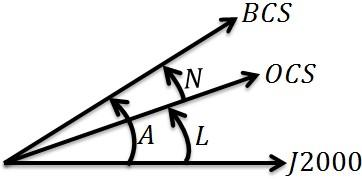Figure 4. Error quaternion in orbital orientation mode

4.1 Calculation of orbital quaternion

The transformation from J2000 to ECEF (WGS) is defined in equation (2) by the transformation matrix $\mathrm{A}_{G I}$. It can be redefined using the quaternion form by the quaternion G which determined by the rotation of the initial quaternion $q_{i n}=\left[\cos \left(\theta_{G 0} / 2\right), 0,0, \sin \left(\theta_{G 0} / 2\right)\right]^{T}$ using the quaternion $q_{r o t G}=\left[\cos \left(\omega_{e} t / 2\right), 0,0, \sin \left(\omega_{e} t / 2\right)\right]^{T}$ as follows

$G=q_{i n} \cdot q_{r o t G}$(33)

The SC state, extracted from GPS data in WGS, consists of the position vector $R=\left[R_{X}, R_{Y}, R_{Z}\right]^{T}$ and the SC velocity vector $V=V_{r}^{G}$ (without taking into account the Earth rotation).The SC velocity vector in WGS frame $V_{a}^{G}$ taking into account the Earth rotation is defined as

$V_{a}^{G}=V_{b}^{G}+V_{r}^{G}$ (34)

$V_{a}^{G}=\omega_{G / I} \times R+V_{r}^{G}$ (35)

where $\omega_{G / I}=\left[0,0, \omega_{e}\right]^{T}$ is Earth mean angular velocity.

## figure_5.jpg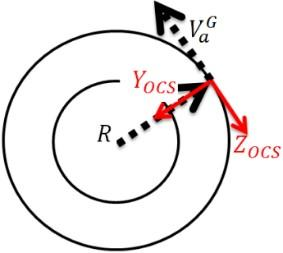Figure 5. SC state vector in OCS in circular orbit

The determination of quaternion Q of transition from OCS to WGS needs two unit vectors measured in the WGS and known in the reference frame OCS. These two unit vectors are the unit vectors of $\mathrm{R}, \mathrm{V}_{\mathrm{a}}^{\mathrm{G}}$ which are measured and calculated respectively, in WGS and known in OCS as $[0,-1,0]$ and $[0,0,-1]$ respectively, as shown in Figure 5. The quaternion  of transition from OCS to WGS is determined as follows

$\hat{\imath}=\frac{u}{|u|}$

$\hat{\jmath}=\frac{(u \times v)}{|u \times v|}$

$\hat{k}=\hat{\imath} \times \hat{\jmath}$ (36)

$M_{W G S}=\left[\hat{\imath}_{W G S} \quad \hat{J}_{W G S} \quad \hat{k}_{W G S}\right]$

$M_{O C S}=\left[\hat{\imath}_{O C S} \hat{\jmath}_{O C S} \hat{k}_{O C S}\right]$

$M_{W G S}=M_{Q} \cdot M_{O C S}$

$M_{Q}=M_{W G S} M_{O C S}^{-1}$

where $M_{O C S}$ must be non-singular. Applying transformation from matrix representation to quaternion representation , the quaternion Q of transition from OCS to WGS can be determined from matrix $M_{Q}$ of transformation from OCS to WGS.

## figure_6.jpg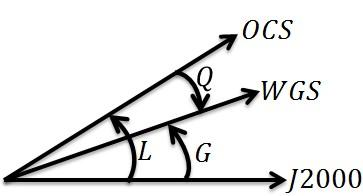Figure 6. Orbital quaternion L

The orbital quaternion L, quaternion of transition from ICS to OCS, as shown in Figure 6, is calculated as follows

$L=G \cdot \tilde{Q}$(37)

4.2 Calculation of SC desired angular rate

The orbital radius $R^{O C S}$, shown in Figure 5, is defined as

$R^{O C S}=\left[0,-\sqrt{R_{X}^{2}+R_{Y}^{2}+R_{Z}^{2}}, 0\right]^{T}$ (38)

The SC velocity vector in WGS frame $V_{a}^{G}$ taking into account the earth rotation is projected into OCS as follows

$V^{O C S}=Q \cdot V_{a}^{G} \cdot \tilde{Q}$ (39)

Considering the $V_{X}^{O C S}$ component to be zero, the desired SC velocity in OCS is $\left[0, V_{Y}^{O C S}, V_{Z}^{O C S}\right]^{T}$. The desired SC orbital angular rate in OCS is $\omega_{\text {orb}}^{O C S}$ defined as

$\omega_{o r b}^{O C S}=\left[\frac{V_{Z}^{O C S}}{R_{Y}^{O C S}}, 0,0\right]^{T}$ (40)

During the orbital orientation mode, the SC motion parameters (linear and orientation) can be determined at the current time instant ($T_C$) based on the SC motion parameters calculated from the latest updated state vectors extracted from GPS data at time instants $\left(T_{0}\right)$ and $\left(T_{P}\right)$. The orbital SC angular acceleration at time instant $\left(T_{0}\right)$ is calculated as follows

$\alpha_{X}^{O C S}=\frac{\omega_{o r b, X}^{O C S}\left(T_{0}\right)-\omega_{o r b, X}^{O C S}\left(T_{P}\right)}{d T_{0 P}}$(41)

The SC acceleration at time instant $\left(T_{0}\right)$ is calculated as

$a^{o c s}=\frac{V^{O C S}\left(T_{0}\right)-V^{O C S}\left(T_{P}\right)}{d T_{0 P}}$ (42)

where $d T_{0 P}=T_{0}-T_{P}$ is the time difference between the latest two available SC state vectors extracted from GPS data. The calculated orbital quaternion $L\left(T_{C}\right)$ at the current time instant $\left(T_{C}\right)$ is determined by rotating the initial orbital quaternion $L\left(T_{0}\right)$ at the time instant $\left(T_{0}\right)$ by the quaternion $q_{r o t L}=\left[\cos \left(\theta_{L} / 2\right), \sin \left(\theta_{L} / 2\right), 0,0\right]^{T}$ as follows

$L\left(T_{C}\right)=L\left(T_{0}\right) \cdot q_{\text {rotL}}$ (43)

where

$\theta_{L}=\omega_{o r b, X}^{O C S}\left(T_{0}\right) d T_{C 0}+\frac{1}{2} \alpha_{X}^{O C S} d T_{C 0}^{2}$ (44)

$d T_{C 0}=T_{C}-T_{0}$ (45)

The desired SC orbital angular rate in OCS at the current time instant $\left(T_{C}\right)$ is $\omega_{\text {orb}}^{O C S}\left(T_{C}\right)$ defined as

$\omega_{o r b}^{O C S}\left(T_{C}\right)=\left[\omega_{o r b, X}^{o c S}\left(T_{0}\right)+\alpha_{X}^{O C S} d T_{C 0}, 0,0\right]^{T}$(46)

Substituting $L\left(T_{C}\right)$ and $\omega_{o r b}^{O C S}\left(T_{C}\right)$ in equations (31), and (32), the error quaternion and the desired SC orbital angular rate projected in BCS are obtained.

5. Target Mode

In this mode, the SC desired orientation is the target frame OCS-N to be tracked. The SC orientation control is performed relative to ICS. The error quaternion $N=q_{e}$ which represents the attitude error between the current orientation A=q and the desired orientation $C=q_{c}$, shown in Figure 7, is defined as

$N=\tilde{C} \cdot A$ (47)

The desired SC angular rate projected in BCS is defined as

$\omega_{C}^{B C S}=\widetilde{N} \cdot \omega_{C}^{O C S-N} \cdot N$ (48)

where $\omega_{C}^{O C S-N}$ is the absolute angular rate (relative to ICS) of the desired target frame (OCS-N) projected in OCS-N.

## figure_7.jpg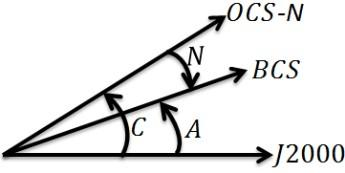Figure 7. Error quaternion in target orientation mode

During imaging (tracking mode), $\omega_{C}^{O C S-N}$ stills constant while the desired orientation quaternion $C=q_{C}$ is updated. This updating requires solving the following kinematic equation of motion

$\dot{q}_{v e c C}=-\frac{1}{2} \Omega\left(\omega_{C}^{O C S-N}\right) q_{v e c C}+\frac{1}{2} q_{4 C} \omega_{C}^{O C S-N}$ (49)

$\dot{q}_{4 C}=-\frac{1}{2}\left(\omega_{C}^{O C S-N}\right)^{T} q_{v e c C}$(50)

where

$\begin{array}{ll}{\Omega\left(\omega_{C}^{O C S-N}\right)} & {} \\ {=\left[\begin{array}{ccc}{0} & {-\omega_{3 C}^{O C S-N}} & {\omega_{2 C}^{O C S-N}} \\ {\omega_{3 C}^{O C S-N}} & {0} & {-\omega_{1 C}^{O C S-N}} \\ {-\omega_{2 C}^{O C S-N}} & {\omega_{1 C}^{O C S-N}} & {0}\end{array}\right]}\end{array}$ (51)

Before the tracking mode and during the SC maneuver toward the sighting point, the SC motion parameters that calculated from the latest updated state vector extracted from GPS data at time instant $\left(T_{0}\right)$ are used as initial conditions to calculate the motion parameters at the starting instant of imaging at time instant $\left(T_{N}\right)$ as described in the following sections.

5.1 Calculation of target position and velocity vectors in WGS

The geodetic coordinate system characterizes a coordinate point near the earth’s surface in terms of longitude, latitude, and height (or altitude), which are respectively denoted by λ, φ, and h. Let a starting point of the on-ground scene pattern is the point P represented in the geodetic system as

$P_{g e o d}=\left[\begin{array}{l}{\lambda} \\ {\varphi} \\ {h}\end{array}\right]$ (52)

The position vector of the starting point of imaging in WGS is $R_{P}^{W G S}$ defined as 

$\mathrm{R}_{P}^{\mathrm{WGS}}=\left[\begin{array}{c}{\left(N_{E}+h\right) \cos \varphi \cos \lambda} \\ {\left(N_{E}+h\right) \cos \varphi \sin \lambda} \\ {\left[N_{E}\left(1-e^{2}\right)+h\right] \sin \varphi}\end{array}\right]$ (53)

where e is the first eccentricity and $N_{E}$ is the prime vertical radius of curvature. These parameters based on the WGS84 are defined as

$e=\frac{\sqrt{R_{E a}^{2}-R_{E b}^{2}}}{R_{E a}}=0.08181919$ (54)

$N_{E}=\frac{R_{E a}}{\sqrt{1-e^{2} \sin ^{2} \varphi}}$ (55)

where the semi-major axis $R_{E a}=6378137[m]$, the semi-minor axis $R_{E b}=R_{E a}(1-f)=6356752[m]$, and the flattening factor $f=1 / 298.257223563$. The velocity vector of the starting point of imaging due to earth rotation velocity in WGS is $V_{P}^{W G S}$ determined as follows

$V_{P}^{W G S}=\omega_{G / I} \times R_{P}^{W G S}$ (56)

5.2 Calculation of target quaternion and desired angular rate

Knowing the estimated time of imaging start $T_{N}$, the quaternion GN shown in Figure 8 (the quaternion of transformation from J2000 to WGS at instant of imaging start) is calculated according to equation (33). The orbital quaternion LN at time instant ($T_N$) is determined by rotating the initial orbital quaternion L at the time instant ($T_0$) by the rotation quaternion $q_{\text {rot} L N}=\left[\cos \left(\theta_{L N} / 2\right), \sin \left(\theta_{L N} / 2\right), 0,0\right]^{T}$ as follows;

$L N\left(T_{N}\right)=L\left(T_{0}\right) \cdot q_{\text {rotLN}}$(57)

where

$\theta_{L N}=\omega_{o r b, X}^{O C S}\left(T_{0}\right) d T_{N 0}+\frac{1}{2} \alpha_{X}^{O C S} d T_{N 0}^{2}$ (58)

$d T_{N 0}=T_{N}-T_{0}$ (59)

## figure_8.jpgFigure 8. Target quaternion CN

The quaternion QN of transition from OCS to WGS at the time of imaging start $T_N$ is determined as follows

$Q N=\widetilde{L N} \cdot G N$ (60)

The desired SC orbital angular rate in OCS at the time instant ($T_N$) is defined as

$\omega_{o r b}^{O C S}\left(T_{N}\right)=\left[\omega_{o r b, X}^{O C S}\left(T_{0}\right)+\alpha_{X}^{O C S} d T_{N 0}, 0,0\right]^{T}$(61)

The orbital radius which represents the position vector of SC in OCS at the time instant ($T_N$) is defined as

R^{\operatorname{ocs}}\left(T_{N}\right)=\left[0, R_{Y}^{O C S}\left(T_{0}\right)+V_{Y}^{O C S}\left(T_{0}\right) d T_{N 0}\right.+\frac{1}{2} a_{Y}^{O C S} d T_{N 0}^{2}, 0 ]^{T} (62) The desired SC velocity in OCS at the time instant (T_N) is defined as \begin{aligned} \operatorname{Vocs}\left(T_{N}\right)=\left[0, V_{Y}^{O C S}\left(T_{0}\right)\right.& \\ &+a_{Y}^{O C S} d T_{N 0}, V_{Z}^{O C S}\left(T_{0}\right) \\ &+a_{Z}^{O C S} d T_{N 0} ]^{T} \end{aligned} (63) The position and velocity vectors of the starting point of the on-ground scene pattern (target), taking into account the earth rotation velocity, are projected into OCS as follows R_{P}^{O C S}=Q N \cdot \mathrm{R}_{P}^{\mathrm{WGS}} \cdot \widetilde{Q N} (64) V_{P}^{O C S}=Q N \cdot \mathrm{V}_{P}^{\mathrm{WGS}} \cdot \widetilde{Q N} (65) The relative position vector between the target and the SC at the time instant (T_N) is defined as R_{r e l}^{O C S}\left(T_{N}\right)=R_{P}^{O C S}-R^{O C S}\left(T_{N}\right) (66) where \left|R_{r e l}^{o c s}\left(T_{N}\right)\right| is the distance from SC center of mass to the earth surface at the target point. The relative velocity vector between the target velocity due to earth rotation (fixed target) and the SC velocity at the target point is defined as V_{r e l}^{O C S}=V_{P}^{O C S}-\left[V^{O C S}\left(T_{N}\right)\right.+\omega_{o r b}^{O C S}\left(T_{N}\right) \times R_{r e l}^{O C S}\left(T_{N}\right) ] (67)

Determination of the quaternion LCN of transition from OCS to OCS-N needs two unit vectors calculated in OCS and known in the reference frame OCS-N. These two unit vectors are the unit vectors of ${R}_{{rel}}^{{OCS}}\left({T}_{N}\right)$ and $\mathrm{V}_{\mathrm{rel}}^{\mathrm{OCS}}$ which are calculated in OCS and considered being in OCS-N in [0,1,0] and [0,0,1] directions, respectively. The quaternion LCN  of transition from OCS to OCS-N is determined as follows

$\hat{\imath}=\frac{u}{|u|}$

$\hat{\jmath}=\frac{(u \times v)}{|u \times v|}$

$\hat{k}=\hat{\imath} \times \hat{\jmath}$

$M_{O C S}=\left[\begin{array}{ll}{\hat{\imath}_{O C S}} & {\hat{\jmath}_{O C S}} & {\hat{k}_{O C S} ]}\end{array}\right.$ (68)

$M_{O C S-N}=\left[\hat{l}_{O C S-N} \quad \hat{\jmath}_{O C S-N} \quad \hat{k}_{O C S-N}\right]$

$M_{O C S}=M_{L C N} \cdot M_{O C S-N}$

$M_{L C N}=M_{O C S} M_{O C S-N}^{-1}$

where $M_{O C S-N}$ must be non-singular. Using the transformation from the matrix representation to quaternion representation , the quaternion LCN of transition from OCS to OCS-N can be determined from the matrix $M_LCN$ of transformation from OCS to OCS-N. The projection of the relative velocity vector $V_rel^OCS$ on OCS-N is determined as follows

$V_{r e l}^{O C S-N}=\widehat{L C N} \cdot V_{r e l}^{O C S} \cdot L C N$ (69)

The relative velocity vector $V_{r e l}^{O C S-N}$ should be perpendicular to the target line of sight. So, it is set to $V_{r e l}^{O C S-N}=\left[V_{r e l, X}^{O C S-N}, 0, V_{r e l, Z}^{O C S-N}\right]$. The projection of the desired SC orbital angular rate on OCS-N is determined as follows

$\omega_{o r b}^{O C S-N}\left(T_{N}\right)=\widehat{L C N} \cdot \omega_{o r b}^{O C S}\left(T_{N}\right) \cdot L C N$ (70)

The relative velocity vector $V_{r e l}^{O C S-N}$ is used to calculate the required pitch retarding angular rate to correct $\omega_{O r b}^{O C S-N}$ in order to increase the image exposer time . Another yaw additional turn rate is added to prevent blurring of image. Both corrections modify the $\omega_{O r b}^{O C S-N}$ before starting of imaging as

$\omega_{C}^{O C S-N}=\omega_{o r b}^{O C S-N}\left(T_{N}\right)+\omega_{\mathrm{corr}}^{O C S-N}$(71)

where $\omega_{\text { corr }}^{O C S-N}$ is the equivalent corrective turn rate including pitch slowing down and yaw rate corresponding to the additional turns. The quaternion of transition from ICS to target frame OCS-N (target quaternion) at the starting instant of imaging is predicted as

$C N=L N \cdot L C N$ (72)

Before the imaging and during the SC maneuver toward the sighting point, the desired SC angular rate $\omega_{C}^{B C S}$ projected in BCS is calculated according to equation (48) using the corrected desired SC angular rate $\omega_{C}^{O C S-N}$ given by equation (71). The instantaneous desired target quaternion C is determined as follows

$C=L \cdot L C N$ (73)

where L is the instantaneous orbital quaternion.

Then the equivalent corrective quaternion ${q}_{corr}$, which includes the pitch slowing down and yaw additional turn, is added as

$C=C \cdot q_{\text {corr}}$ (74)

In addition to any corrective quaternion may be set from the control ground station.

During imaging, $\omega_{C}^{O C S-N}$ is maintained constant at its value at the starting instant of imaging while the desired orientation quaternion C is updated as illustrated in equations (49) to (51).

6. Practical Case Study

For the rigid SC under consideration, the nominal inertia matrix in units of $\left[K g \cdot m^{2}\right]$ is

$J=\left[\begin{array}{ccc}{430} & {-2} & {4} \\ {-2} & {250} & {3} \\ {4} & {3} & {425}\end{array}\right]$ (75)

The SC state vector can be extracted from the given two line element (TLE)

1 29283U 06022G 06177.28732010 .00766286 10823-4 13334-2 0 101

2 29283 51.5595 213.7903 0202579 95.2503 267.9010 15.73823839 1061 (76)

Taking damping ratio $\zeta \approx 0.9$  and the natural frequency $\omega_{n} \approx 0.45[r a d / s]$  yields the positive scalars $k \approx 0.4\left[s^{-2}\right]$  and $d \approx 0.8\left[s^{-1}\right]$ .

The maximum angular rate $\left|\omega_{i}\right|_{\max }$  is assumed to be 85% of the high accuracy range $(3[\operatorname{deg} / s])$  of measuring angular velocity using star trackers. The maximum saturation torque limits in each control channel $U=(1,0.5,1)[\mathrm{N} . \mathrm{m}]$ .

The maximum control acceleration in the maneuver direction $a_{m a x p}$  is chosen to be 60% of allowable limit to accommodate the nonlinear nature of quaternion-based phase-plane dynamics, actuator dynamics, and control acceleration uncertainty. The simulation is carried out using time-optimal nonlinear three-axis quaternion feedback control logic.

The simulation is performed for realistic mission with many closely placed ground targets. The mission plan includes: normal scene imaging (twice) and stereo pair imaging (consists of two scenes) with the following Euler angles sets; [0; -4; -22], [20; -4; -18], [-25; -3; -2], and [25; -3; -1] [deg] .

## figure_9.jpg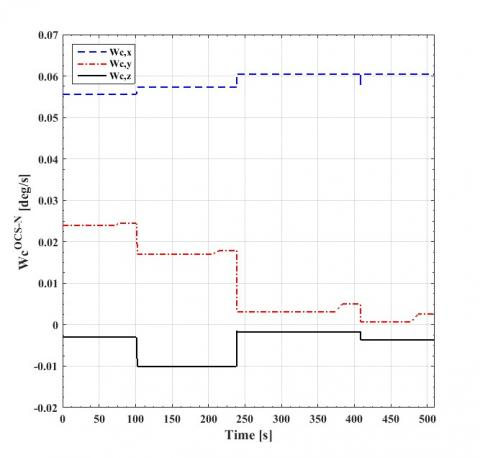Figure 9. Corrected desired SC angular rate projected on OCS-N

## figure_10.jpg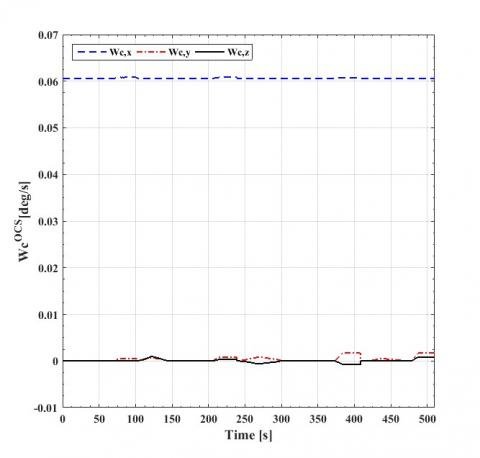Figure 10. Corrected desired SC angular rate projected on OCS

Figure 9 shows the corrected desired SC angular rate projected on OCS-N corresponding to the mentioned multi-target mode. The applied corrective rate in this case study is in yaw channel with zero corrective rates in pitch channel (no need to increase the exposer time/design aspect). In Figure 9, the correction clearly appears in $\omega_{C, Y}^{O C S-N}$  yaw channel (red, dash-dot line) just before and during each scene then the value of $\omega_{C}^{O C S-N}$  jump to the next target value.

Figure 10 shows the corrected desired SC angular rate projected on OCS where the dominant component is $\omega_{\text { orb }, \mathrm{X}}^{\mathrm{OCS}}$  in pitch channel due to SC orbital movement.

## figure_11.jpg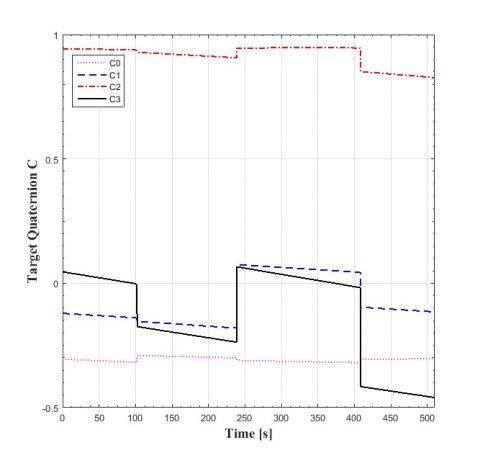Figure 11. Target quaternion relative to ICS

Figure 11 shows the target quaternion C, calculated using equation (73), then corrected according to equation (74) before imaging start, and propagated using equations (49) to (51) during the imaging.

7. Euler Angles Representation and Attitude Errors Calculation

For Euler angles representation, the SC desired orientation relative to OCS is represented in Figure 12 by the quaternion LC of transition from OCS to OCS-N. Also the actual SC orientation relative to OCS is represented in Figure 13 by the quaternion LB of transition from OCS to BCS. These quaternions are calculated according to the following equations (extracted from Figure 14) as

$L C=\tilde{L} \cdot C$(77)

$L B=L C \cdot N \quad$ or $L B=\tilde{L} \cdot A$ (78)

where A is the current attitude quaternion relative to ICS.

The quaternions LC and LB can be converted to EA(LC) and EA(LB)  respectively where EA(*)  is the Euler angles representation for attitude quaternion (*) defined by  rotation sequence (3-1-2) Roll, Pitch, and Yaw angles (EA=$[\text { Pitch, Yaw, Roll }]^{\mathrm{T}}$) . Figure 15 and Figure 16 illustrate the Euler angles representation for desired and actual SC orientation respectively corresponding to this multi-target mode.

The SC absolute angular rate projected on the BCS ($\omega^{\mathrm{BCS}}$) corresponding to this multi-target mode is illustrated in Figure 17. This figure illustrates that SC angular rates during the three maneuvers did not reach to maximum angular rate $\left|\omega_{i}\right|_{\max }$.

The SC absolute angular rate is represented via Euler rate by projection on OCS as illustrated in Figure 18 using the following equation

$\overrightarrow{E A}=[\dot{P}, \dot{Y}, \dot{R}]^{T}=\omega^{O C S}=L B \cdot \omega^{B C S} \cdot \widetilde{L B}$ (79)

## figure_12.jpgFigure 12. Target quaternion relative to OCS (quaternion LC)

## figure_13.jpg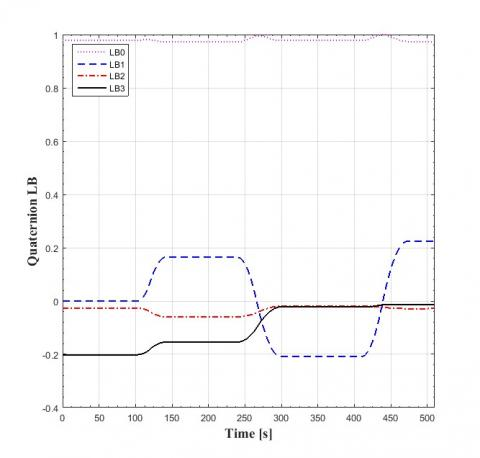Figure 13. SC actual orientation relative to OCS (quaternion LB)

## figure_14.jpg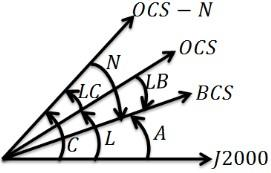Figure 14. LC and LB quaternions

## figure_15.jpg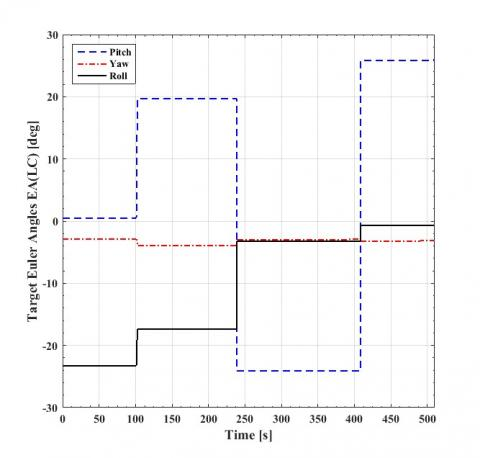Figure 15. Euler angles representation for desired SC orientation

## figure_16.jpgFigure 16. Euler angles representation for actual SC orientation

## figure_17.jpg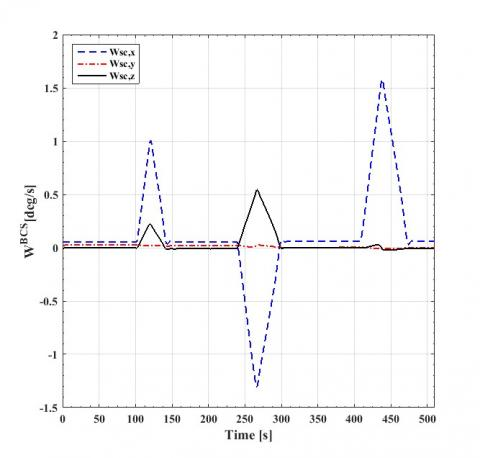Figure 17. SC absolute angular rate projected on the BCS

## figure_18.jpg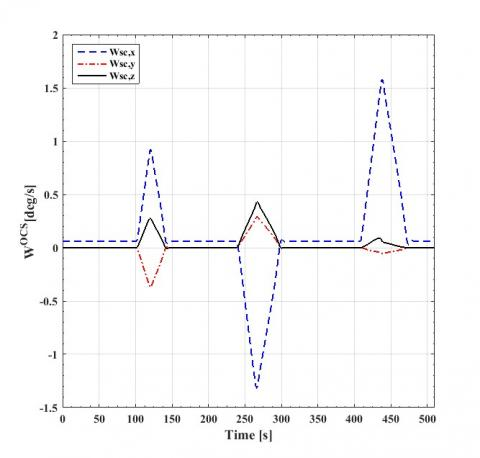Figure 18. SC absolute angular rate projected on the OCS

## figure_19.jpg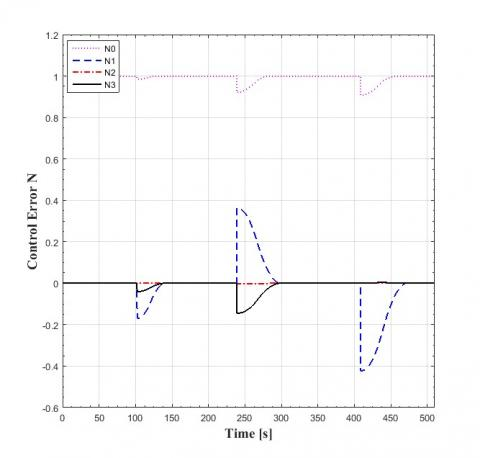Figure 19. Control error

## figure_20.jpg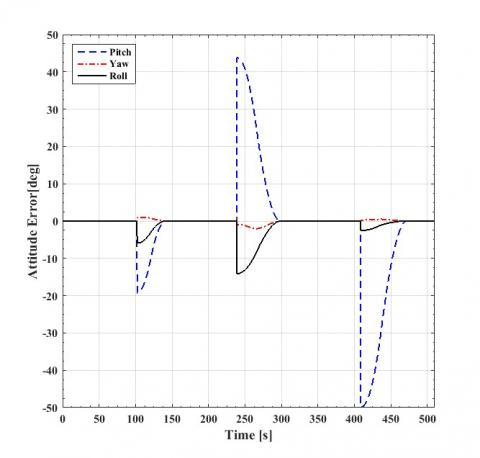Figure 20. Attitude error

Considering ideal attitude determination sensors, the control error shown in Figure 19 is determined as the difference between target (desired) attitude and estimated attitude. The attitude error/pointing accuracy shown in Figure 20 is determined as the difference between the current and target (desired) attitude as follows

$A_{e}=E A(L B)-E A(L C)$ or $A_{e}=E A(N)$ (80)

The overall attitude error during multi-target mode period is illustrated in Figure 20.

Figures 20 to 22 make zoom in the stabilization period before 2nd scene imaging. Figure 22 shows the attitude error/pointing accuracy in the three channels (pitch, yaw, and roll) and illustrates that the largest error is in pitch channel. Figure 23 illustrates that, the mean value of attitude error in pitch channel is $A_{\mathrm{e}}=-0.006094[\mathrm{deg}]=-0.366[\mathrm{arcmin}]$ and the stability (peak to peak motion) is $S_e=0.000712[\operatorname{deg}]=0.043[\text { arcmin }]$. These values are within the required 0.05[deg] accuracy and 0.0167[deg] stability.

## figure_21.jpg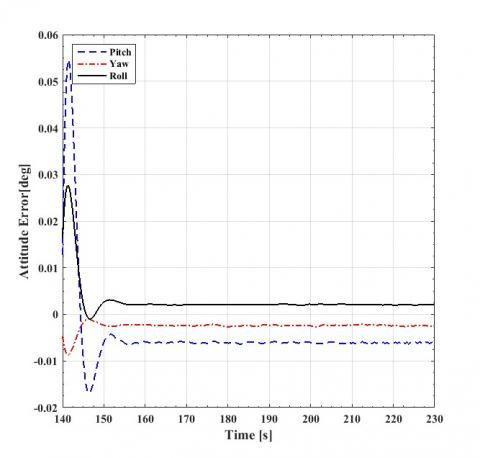Figure 21. Zoom in attitude error from 140:230[s]

## figure_22.jpg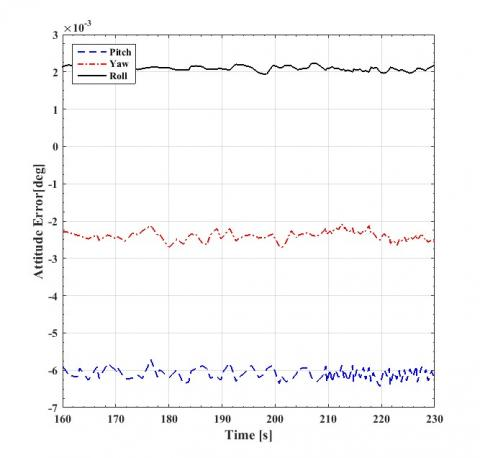Figure 22. Zoom in attitude error from 160:230[s]

## figure_23.jpg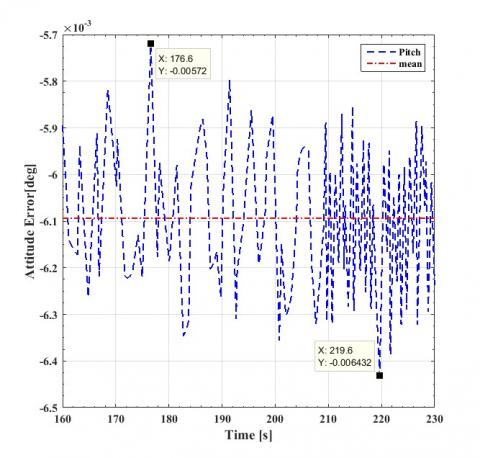Figure 23. Attitude error in pitch channel from 160:230[s]

## figure_24.jpg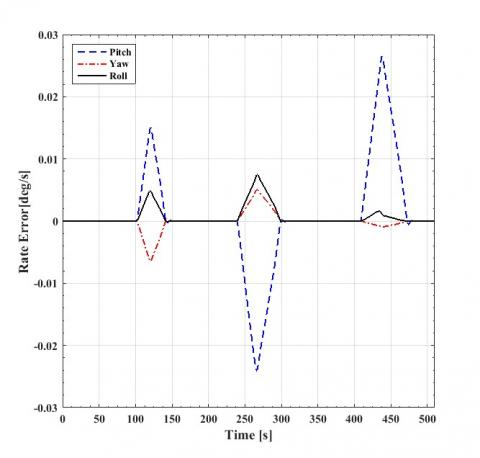Figure 24. Rate error

## figure_25.jpgFigure 25. Zoom in rate error from 150:230[s]

## figure_26.jpg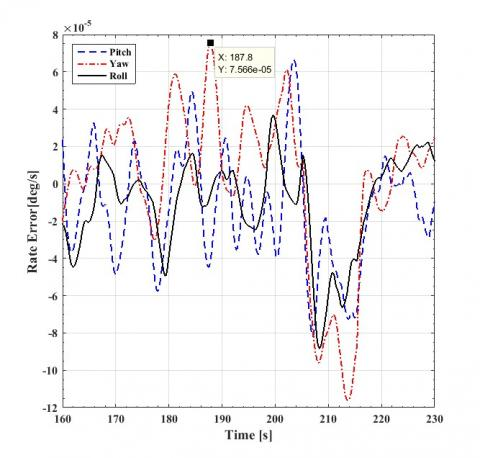Figure 26. Zoom in rate error from 160:230[s]

The SC Euler rate error during multi-target mode is shown in Figure 24 to Figure 26. The SC Euler rate error is determined as the difference between the true (current) rate and target (desired) rate on OCS as

$\dot{E A}_{e}=[\dot{R}, \dot{P}, \dot{Y}]^{T}=\omega^{O C S}-\omega_{C}^{O C S}$ (81)

The overall rate error during multi-target mode period is illustrated in Figure 24. Figure 25 and Figure 26 make zoom in the stabilization period before 2nd scene imaging. Figure 26 illustrates that, the angular rate error is not worse than 0.0001$[\mathrm{deg} / \mathrm{s}]$  which is within the allowable 0.001$[\mathrm{deg} / \mathrm{s}]$  rate error.

8. Controller Limits Comparison

Eigen-axis outer ellipsoid, Eigen-axis inscribed ellipsoid, independent axes outer ellipsoid, and independent axes inscribed ellipsoid are different limits used for the controller output torque u . The SC angular rate stabilization time for some dominant maneuver directions are determined for these limits. Table 2 shows one of these simulations results for desired rotation angles equals 10*[0.9239; 0; 0.3827] [deg]. In general, it can be concluded that, using the inscribed ellipsoid either with Eigen-axis or independent limits reduces the capability and tends to slow orientation. Using the Eigen-axis outer ellipsoid increases the used capability while the Eigen-axis is maintained.

Table 2. Stabilization time for different controller limit

 controller output torque limit stabilization time [sec] Eigen-axis 0.75$U_{e}$ (Inscribed ellipsoid) 42.22 $U_{e}$ (Outer ellipsoid) 31.06 Independent axes U  (Outer ellipsoid) 31.26 0.75$U$  (Inscribed ellipsoid) 40.19
9. Conclusions

A complete kinematics of fixed ground target tracking has been presented. The desired SC velocity and angular rate are defined in OCS. The target quaternion is updated during imaging. The SC motion parameters at the starting instant of imaging are calculated during the SC maneuver toward the sighting point. The position and velocity vectors for each of target and SC at the imaging start instant are determined in OCS and the relative position and velocity vectors are determined. The quaternion of transition from OCS to OCS-N is calculated using these relative vectors. The projection of the relative velocity vector and the desired SC angular rate on OCS-N are determined. The relative velocity vector is used to calculate the required pitch retarding angular rate to correct the desired angular rate in order to increase the image exposer time. The non-linear tracking control algorithm presented in  by Wie is modified and tolerated to guarantee the largest magnitudes of body acceleration along the worst direction. The proposed controller cares about fast ground-target tracking. The simulation results also show well and considerable robustness behavior of the proposed controller. The results ensure that pointing accuracy, stability, and angular rate error in three channels are within the allowable 0.05 [deg], 0.0167[deg], and 0.001[deg/s]  limits, respectively. Momentum monitoring ensures that the system is kept well away below the permissible limits.

References

 Wie B, Bailey D, Heiberg C. (2002). Rapid multitarget acquisition and pointing control of agile spacecraft. Journal of Guidance, Control, and Dynamics 25(1): 96-104. https://doi.org/10.2514/2.4854

 Cao X, Yue C, Liu M, Wu B. (2016). Time efficient spacecraft maneuver using constrained torque distribution. Acta Astronautica 123: 320-329. https://doi.org/10.1016/j.actaastro.2016.03.026

 Markley FL, Crassidis JL. (2014). Fundamentals of spacecraft attitude determination and control. New York: Springer 33. https://doi.org/10.1007/978-1-4939-0802-8

 Zhang X, Liu X. (2014). Chattering free adaptive sliding mode control for attitude tracking of spacecraft with external disturbance. In Proceedings of the 33rd Chinese Control Conference. IEEE, pp. 2224-2228. https://doi.org/10.1109/ChiCC.2014.6896977

 De Ruiter A, Damaren C, Forbes J. (2012). Spacecraft Dynamics and Control: An Introduction. John Wiley & Sons.

 Ali A, Elsheikh G, Hegazy M. (2017). Coupled spacecraft orbital and attitude modeling and simulation in multi-complex modes. world academy of science, engineering and technology. International Journal of Mechanical, Aerospace, Industrial, Mechatronic and Manufacturing Engineering 11(2): 374-385. https://doi.org/10.5281/zenodo.1128897

 Sugita M. (2017). Torque distribution algorithm for effective use of reaction wheel torques and angular momentums. Acta Astronautica 139: 18-23. https://doi.org/10.1016/j.actaastro.2017.06.014

 King J, Karpenko M. (2016). A simple approach for predicting time-optimal slew capability. Acta Astronautica 120: 159-170. https://doi.org/10.1016/j.actaastro.2015.12.009

 Marsh H, Karpenko M, Gong Q. (2016). Energy constrained shortest-time maneuvers for reaction wheel satellites. In AIAA/AAS Astrodynamics Specialist Conference, pp. 5579. https://doi.org/10.2514/6.2016-5579

 Koh D, AlSayegh A, Lee H. (2016). In-orbit performance of attitude control system in DubaiSat-2. In 14th International Conference on Space Operations, p. 2600. https://doi.org/10.2514/6.2016-2600

 Xin X, Li Z, Liu X, Chen Z. (2015). Near minimum-time feedback attitude control with multiple saturation constraints for satellites. In 2015 34th Chinese Control Conference (CCC). IEEE, pp. 1031-1036. https://doi.org/10.1109/ChiCC.2015.7259775

 Bailey D. (2002). Control of Velocity Limited Systems. US 6,477,433 B1.

 Cai G, Chen B, Lee T. (2011). Unmanned rotorcraft systems. Springer Science & Business Media. https://doi.org/10.1007/978-0-85729-635-1

 Vuuren G. (2015). The design and simulation analysis of an attitude determination and control system for a small earth observation satellite. Doctoral Dissertation, Stellenbosch University, Stellenbosch, South Africa. https://doi.org/10.1.1.1027.9559